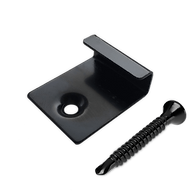top of page## ​

Number of decking boards per m2 = 1.72

Example: 20m2 deck would be 20 x 1.72 = 34.4 so you would need 35 decking boards PLUS a further 5% on for offcuts or wastage.

Check your sums! Many people think a 5 x 5 deck is 5m2, it is not, it would be 25m2. Width x Length = m2.

If you need to work out the m2 from the number of decking boards, divide by 1.72.

Example: 100 decking boards / 1.72 = 58m2 deck.

REMEMBER TO ADD 5% FOR WASTAGE## FASTENERS

You will need: 1 bag per 5m2 for 6mm fasteners. For example a 25m2 deck would need 5 bags. 25/5 = 5.

PLUS you will need: 1 bag per 25m2 for starter fasteners. For example a 36m2 deck would need 2 bags of starter fasteners. 36/25 = 1.44.## FASCIA, TRIM & JOISTS

Fascia boards and corner trims depend on the perimeter size of your deck. Each length is 2.2m.

Example: A 4x5 deck would be 18 linear meters perimeter.

18/2.2 = 9 lengths.

DECKING CALCULATOR
bottom of page